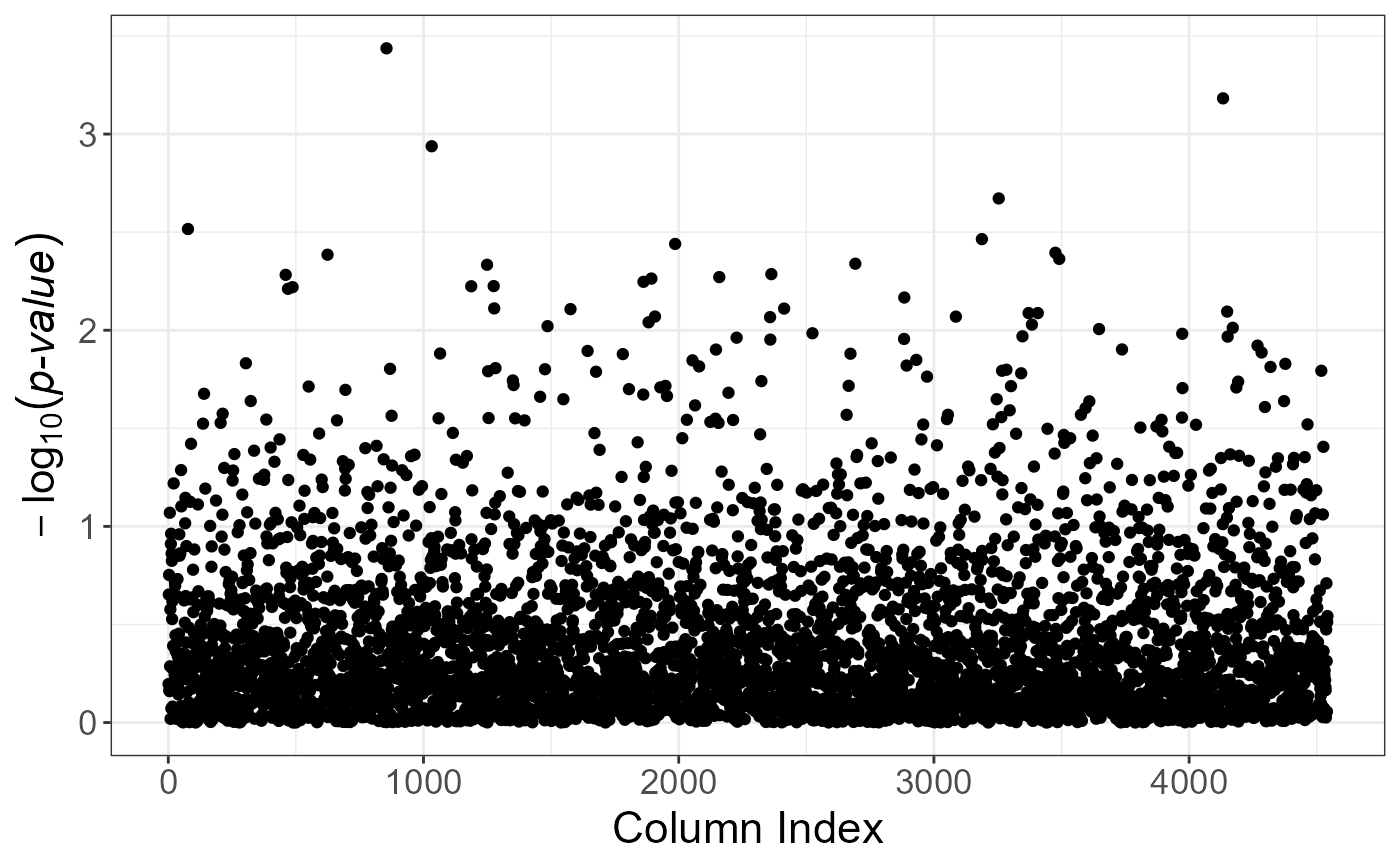Plot method for class mhtest.

# S3 method for mhtest
plot(x, type = c("hist", "Manhattan", "Q-Q", "Volcano"), coeff = 1, ...)

## Arguments

x An object of class mhtest. Either. "hist": histogram of p-values (the default). "Manhattan": plot of the negative logarithm (in base 10) of p-values. "Q-Q": Q-Q plot. "Volcaco": plot of the negative logarithm of p-values against the estimation of coefficients (e.g. betas in linear regression) Relative size of text. Default is 1. Not used.

## Value

A ggplot2 object. You can plot it using the print method. You can modify it as you wish by adding layers. You might want to read this chapter to get more familiar with the package ggplot2.

set.seed(1)
plot(test)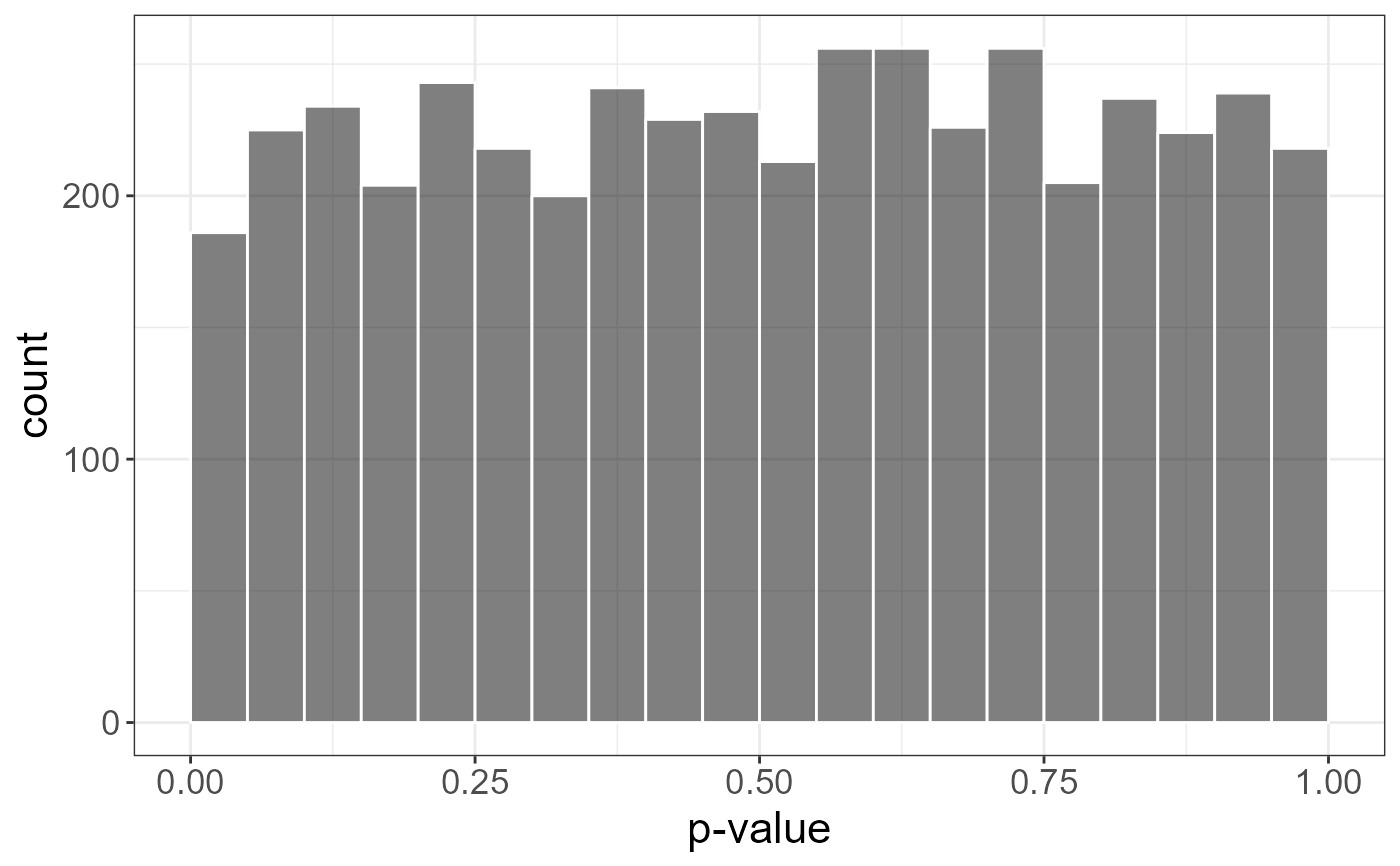plot(test, type = "Volcano")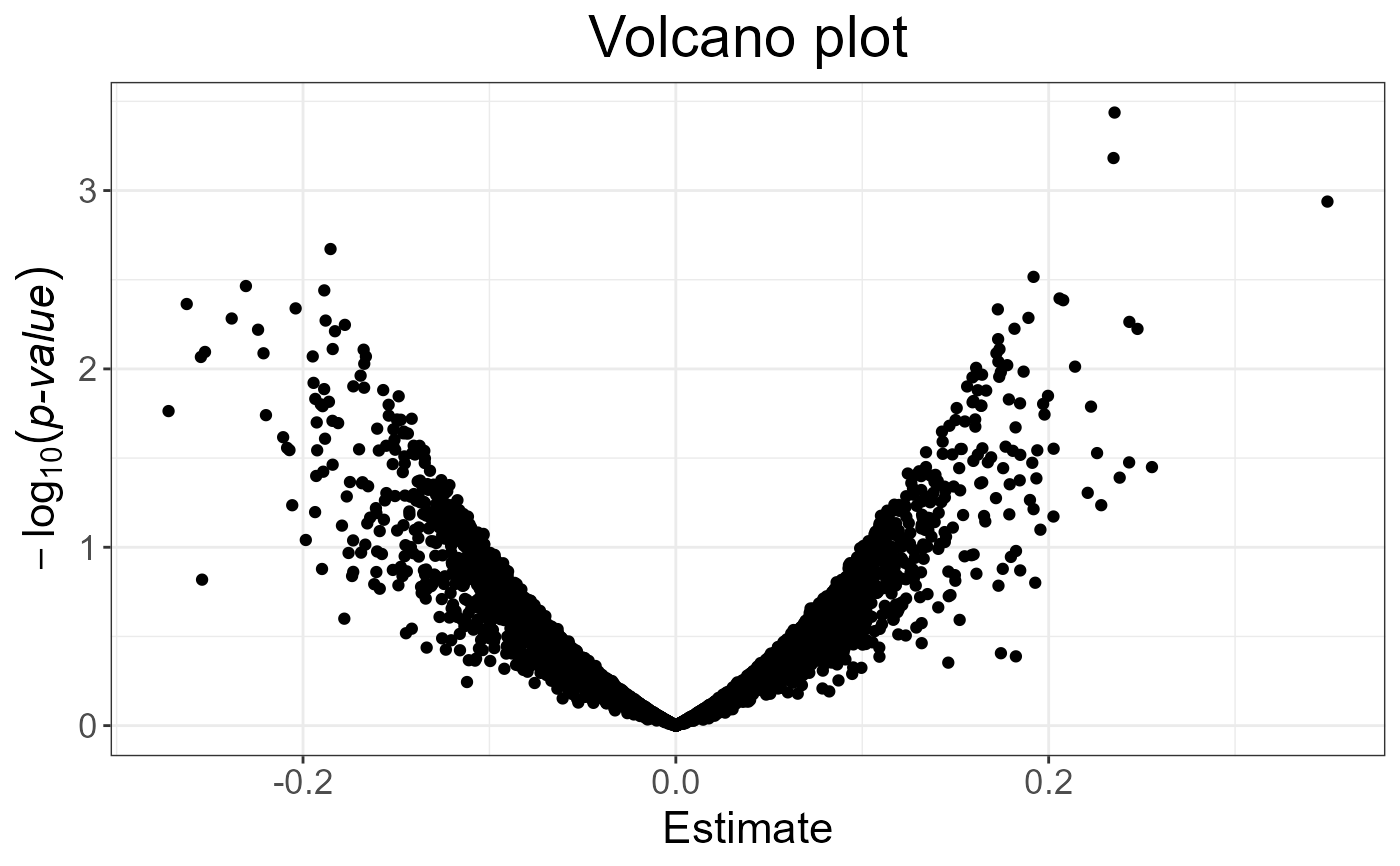plot(test, type = "Q-Q")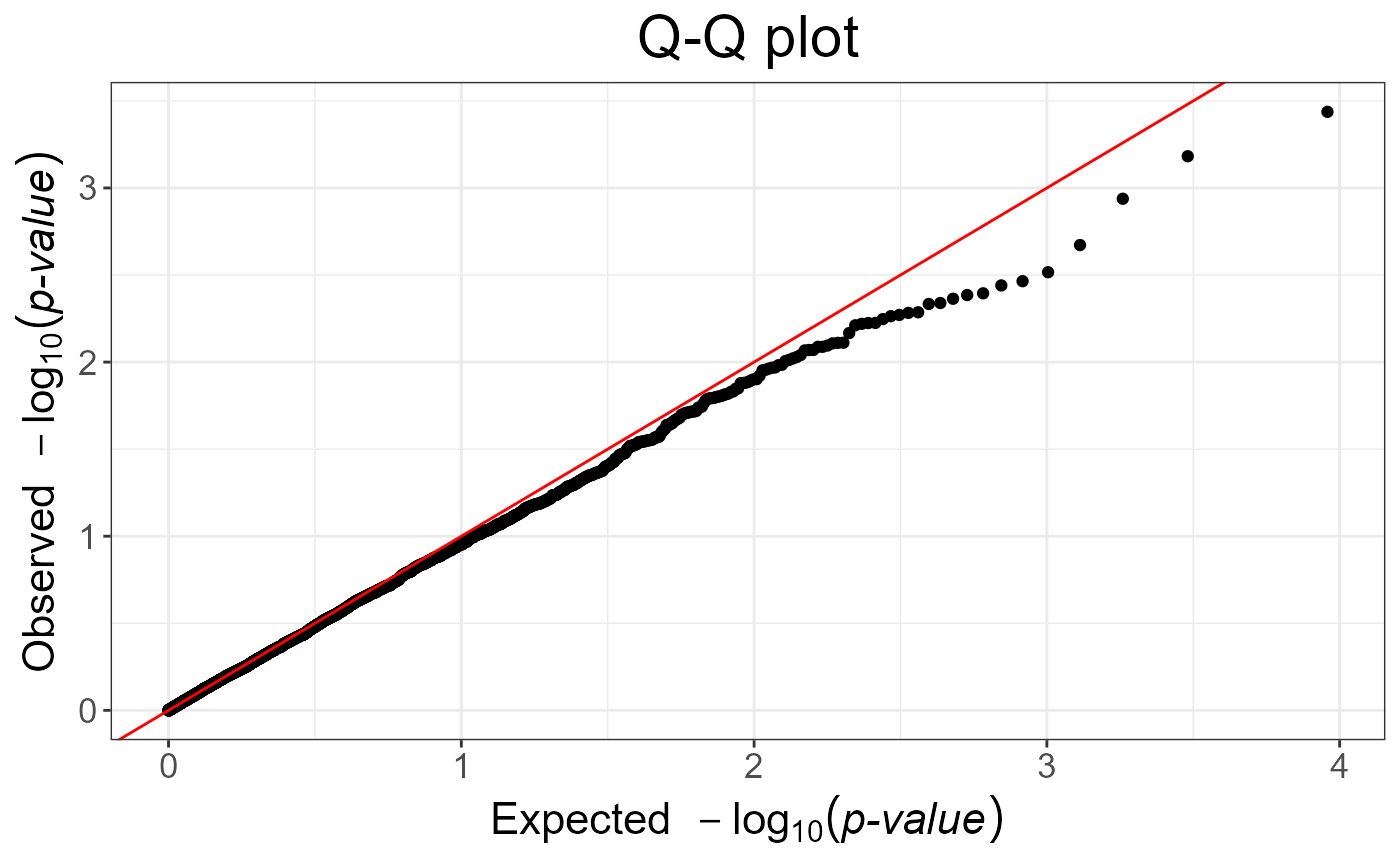plot(test, type = "Manhattan")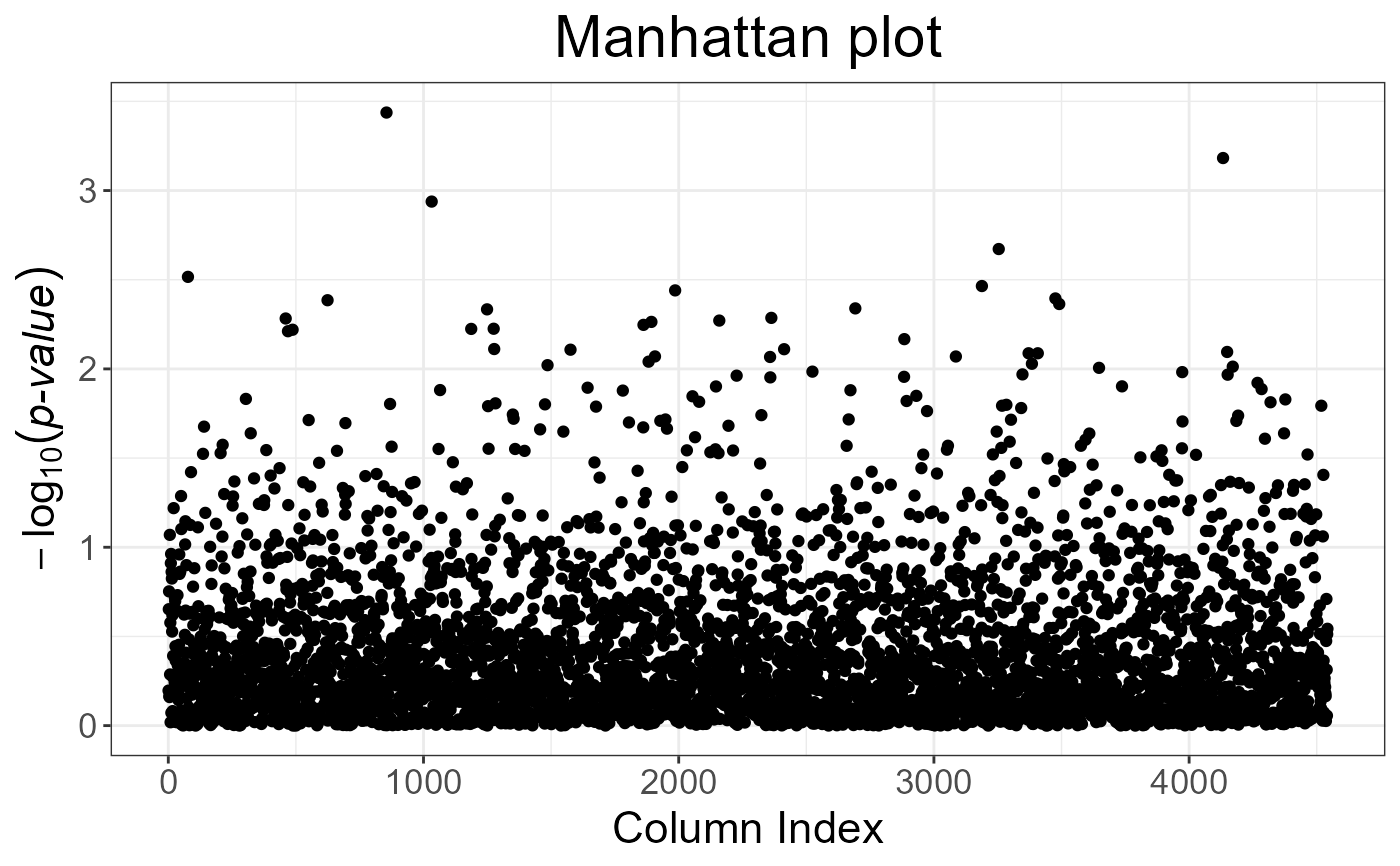plot(test, type = "Manhattan") + ggplot2::ggtitle(NULL)